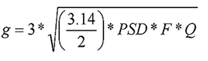Miles Equation - Vibration

The following Miles equation calculates a quas-static acceleration which simulates the 3σ acceleration at a given frequency:where:

g = the equivalent quasi-static acceleration

3 = 3σ Raleigh distribution

PSD = the power spectral density at the resonant frequency

Q = the transmissibility at resonance (typically square root natural frequency)

F = the resonant frequency

TRANSMISSIBILITY, Q

The transmissibility, Q, of a component can be conservatively estimated to be the square root of its natural frequency. Typical values range from 15 to 25 depending on the application. Real values can only be obtained through vibe testing.

APPLYING G LOADING TO FEA (BASED ON METHOD I)

From the Mile's equation, the resulting quasi-static acceleration is applied as a static body load in a finite element model (FEM) of the component. In the FEMAP preprocessor, the 3s g loading should be multiplied by gravity, 386.4 (in/sec2), to convert it into an acceleration loading.© Copyright 2000 - 2019, by Engineers Edge, LLC www.engineersedge.comMembership Register | LoginHomeEngineering Book StoreEngineering ForumExcel App. DownloadsOnline Books & ManualsEngineering NewsEngineering VideosEngineering CalculatorsEngineering ToolboxGD&T Training Geometric Dimensioning TolerancingDFM DFA TrainingTraining Online EngineeringAdvertising CenterCopyright Notice